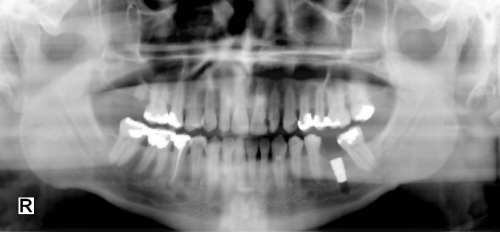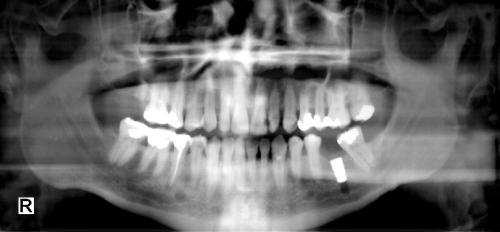﻿ SubtractBackgroundCommand Class | Leadtools.ImageProcessing.Core | Raster, Medical, Document Help
←Select platform
In This Topic ▼

# SubtractBackgroundCommand Class

Summary
Removes the background from the image.
Syntax
C#
Objective-C
C++/CLI
Java
``public class SubtractBackgroundCommand : RasterCommand ``
``@interface LTSubtractBackgroundCommand : LTRasterCommand ``
````public class SubtractBackgroundCommand `
`    extends RasterCommand ````
``public ref class SubtractBackgroundCommand : public RasterCommand   ``
Remarks
• This command is useful, especially with medical images and grayscale images in correcting non-uniform brightness.
• The rolling ball algorithm works as follows:

1. Consider the image to be a 3-D surface and the z-axis to be the intensity of the image [The component V from the HSV color space].
2. Roll a 3-D ball beneath the surface so all the points of the ball are under the surface with one or more points of the ball tangent to the surface.
3. The tangent points to the rolling ball are considered to be the background.
4. Subtract the background from the original image.
• The Rolling Ball Radius should be at least as large as the radius of the largest object in the image that is not part of the background to ensure the separation of the background from any objects.

• A small radius allows the detection of small objects, whereas a larger radius will detect both small and large objects.
• When subtracting the background, sometimes the result is dim. In such a case you can enhance the brightness after subtracting the background by using the brightness factor value, which functions similar to MultiplyCommand. Passing 100 for the brightness factor leaves the brightness unchanged.
• This command supports 12 and 16-bit grayscale and 48 and 64-bit color images. Support for 12 and 16-bit grayscale and 48 and 64-bit color images is available only in the Document/Medical toolkits.
• This command does not support signed data images.
• This command does not support 32-bit grayscale images.

Subtract Background Function - BeforeSubtract Background Function - AfterView additional platform support for this Subtract Background function.

Example

Run the SubtractBackgroundCommand on an image.

C#
````using Leadtools; `
`using Leadtools.ImageProcessing; `
`using Leadtools.Codecs; `
`using Leadtools.ImageProcessing.Core; `
` `
`public void SubtractBackgroundCommandExample() `
`{ `
`   // Load an image `
`   RasterCodecs codecs = new RasterCodecs(); `
`   codecs.ThrowExceptionsOnInvalidImages = true; `
` `
`   RasterImage image = codecs.Load(Path.Combine(LEAD_VARS.ImagesDir, @"ImageProcessingDemo\Image4.Tif")); `
` `
`   // Prepare the command `
`   SubtractBackgroundCommand command = new SubtractBackgroundCommand(); `
`   command.RollingBall = 50; `
`   command.BrightnessFactor = 100; `
`   command.ShrinkingSize = SubtractBackgroundCommandType.DependOnRollingBallSize; `
`   command.Flags = SubtractBackgroundCommandFlags.BackgroundIsDarker; `
`   //Apply Subtract Background effect on the image. `
`   command.Run(image); `
` `
`} `
` `
`static class LEAD_VARS `
`{ `
`   public const string ImagesDir = @"C:\LEADTOOLS22\Resources\Images"; `
`} ````
Requirements
Help Version 22.0.2023.1.25